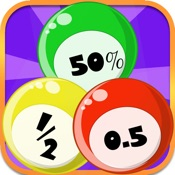Percent Bingo helps you practice Grade 3,4,5 Math - Lumos Educational App StorePrice -\$2.57

DESCRIPTION:

" Percent Bingo...is just what you are looking for, an engaging and easy way to build fluency... " -- TeachersWithApps.com 5/5 Stars - Edu. App Certified -- EducationalAppStore.com Fluency Games is proud to present their newest game, Percent Bingo! Play the classic bingo game designed to build fluency and number sense with fractions, percents and decimals. Bingo numbers are given as fractions or decimals and the player converts them to percents before placing on the board. Percent Bingo features adaptive play, and the game introduces more difficulty fractions as the playe

OVERVIEW:

Percent Bingo is a free educational mobile app By Marty Esterman.It helps students in grades 3,4,5 practice the following standards 3.NF.3,4.NF.C.5,5.NF.B.3.

This page not only allows students and teachers download Percent Bingo but also find engaging Sample Questions, Videos, Pins, Worksheets, Books related to the following topics.

1. 3.NF.3 : Explain equivalence of fractions in special cases, and compare fractions by reasoning about their size. (Grade 3 expectations in this domain are limited to fractions with denominators 2, 3, 4, 6, and 8.).

2. 4.NF.C.5 : Express a fraction with denominator 10 as an equivalent fraction with denominator 100, and use this technique to add two fractions with respective denominators 10 and 100. For example, express 3/10 as 30/100 and add 3/10 + 4/100 = 34/100. (Students who can generate equivalent fractions can develop strategies for adding fractions with unlike denominators in general. But addition and subtraction with unlike denominators in general is not a requirement at this grade.) (Grade 4 expectations in this domain are limited to fractions with denominators 2, 3, 4, 5, 6, 8, 10, 12, and 100.).

3. 5.NF.B.3 : Interpret a fraction as division of the numerator by the denominator (a/b = a ÷ b). Solve word problems involving division of whole numbers leading to answers in the form of fractions or mixed numbers, e.g., by using visual fraction models or equations to represent the problem. For example, interpret 3/4 as the result of dividing 3 by 4, noting that 3/4 multiplied by 4 equals 3 and that when 3 wholes are shared equally among 4 people each person has a share of size 3/4. If 9 people want to share a 50-pound sack of rice equally by weight, how many pounds of rice should each person get? Between what two whole numbers does your answer lie?.

3
4
5

STANDARDS:

3.NF.3
4.NF.C.5
5.NF.B.3

Software Version: 1.2.0

Category: Education

RELATED APPS

Rate this App?
0

0 Ratings & 0 Reviews

5
0
0
4
0
0
3
0
0
2
0
0
1
0
0EdSearch WebSearch